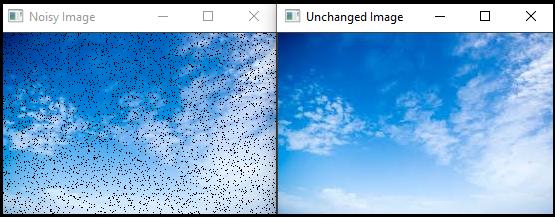# How to change Pixel values using Direct Access Method in OpenCV?

In the previous method (the 'at' method), we need to specify the image type while accessing the pixel values. There is another method which is simpler than 'at' method. It is called direct access method. To access pixel value using this method, we need to specify the Mat type such as Mat<uchar>, Mat<Vec3b>, Mat<Vec2i> and so on.

The following program demonstrates how to change Pixel Values using Direct Access Method in OpenCV.

## Example

#include<iostream>
#include<opencv2/highgui/highgui.hpp>
using namespace cv;//Declaring cv namespace
using namespace std;
void direct_access(Mat_<Vec3b> &image, int n){ //Declaring the function//
for (int x = 0; x < n; x++){ //initiating a for loop//
int i = rand() % image.cols;//accessing random column//
int j = rand() % image.rows;//accessing random rows//
image(j, i) = 0;//setting the pixel values to zero//
}
}
int main() {
Mat_<Vec3b> image;//taking an image matrix//
Mat unchanged_Image;//taking another image matrix//
namedWindow("Noisy Image");//Declaring an window//
namedWindow("Unchanged Image");//Declaring another window//
direct_access(image, 4000);//calling the direct access function//
imshow("Noisy Image", image);//showing the Noisy image
imshow("Unchanged Image", unchanged_Image);//showing the unchanged image//
waitKey(0);//wait for Keystroke//
return 0;
}

## Output